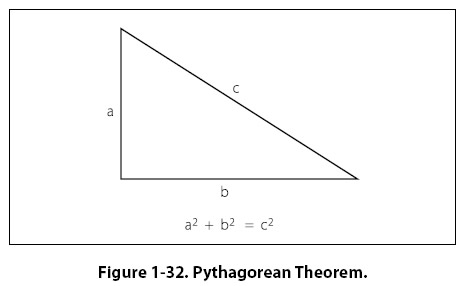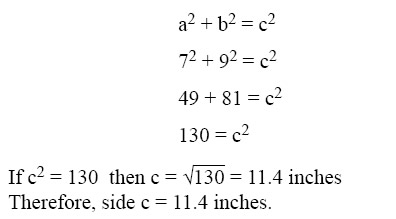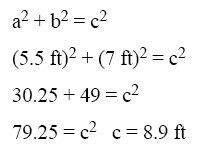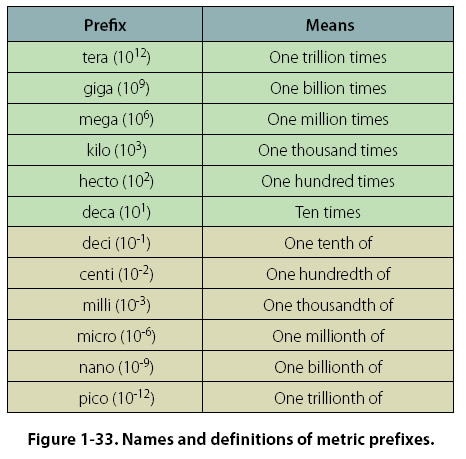Pythagorean Theorem The Pythagorean Theorem is named after the ancient Greek mathematician, Pythagoras (~500 B.C.). This theorem is used to find the third side of any right triangle when two sides are known. The Pythagorean Theorem states that a2 + b2 = c2. [Figure 1-32] Where c = the hypotenuse of a right triangle, a is one side of the triangle and b is the other side of the triangle.Example: What is the length of the longest side of a right triangle, given the other sides are 7 inches and 9 inches? The longest side of a right triangle is always side c, the hypotenuse. Use the Pythagorean Theorem to solve for the length of side c as follows:Example: The cargo door opening in a military airplane is a rectangle that is 5 1/2 feet tall by 7 feet wide. A section of square steel plate that is 8 feet wide by 8 feet tall by 1 inch thick must fit inside the airplane. Can the square section of steel plate fit through the cargo door? It is obvious that the square steel plate will not fit horizontally through the cargo door. The steel plate is 8 feet wide and the cargo door is only 7 feet wide. However, if the steel plate is tilted diagonally, will it fit through the cargo door opening? The diagonal distance across the cargo door opening can be calculated using the Pythagorean Theorem where “a" is the cargo door height, “b" is the cargo door width, and “c" is the diagonal distance across the cargo door opening.The diagonal distance across the cargo door opening is 8.9 feet, so the 8-foot wide square steel plate will fit diagonally through the cargo door opening and into the airplane. Measurement Systems Conventional (U.S. or English) System Our conventional (U.S. or English) system of measurement is part of our cultural heritage from the days when the thirteen colonies were under British rule. It started as a collection of Anglo-Saxon, Roman, and Norman-French weights and measures. For example, the inch represents the width of the thumb and the foot is from the length of the human foot. Tradition holds that King Henry I decreed that the yard should be the distance from the tip of his nose to the end of his thumb. Since medieval times, commissions appointed by various English monarchs have reduced the chaos of measurement by setting specific standards for some of the most important units. Some of the conventional units of measure are: inches, feet, yards, miles, ounces, pints, gallons, and pounds. Because the conventional system was not set up systematically, it contains a random collection of conversions. For example, 1 mile = 5,280 feet and 1 foot = 12 inches. Metric System The metric system, also known as the International System of Units (SI), is the dominant language of measurement used today. Its standardization and decimal features make it well-suited for engineering Figure 1-32. Pythagorean Theorem. and aviation work. The metric system was first envisioned by Gabriel Mouton, Vicar of St. Paul’s Church in Lyons, France. The meter is the unit of length in the metric system, and it is equal to one ten-millionth of the distance from the equator to the North Pole. The liter is the unit of volume and is equal to one cubic decimeter. The gram is the unit of mass and is equal to one cubic centimeter of water. All of the metric units follow a consistent naming scheme, which consists of attaching a prefix to the unit. For example, since kilo stands for 1,000 one kilometer equals 1,000 meters. Centi is the prefix for one hundredth, so one meter equals one hundred centimeters. Milli is the prefix for one thousandths and one gram equals one thousand milligrams. Refer to Figure 1-33 for the names and definitions of metric prefixes.©AvStop Online Magazine                                                                                                                                                      Contact Us              Return To Books